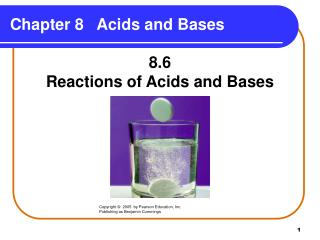DownloadDownload PresentationChapter 8 Acids and Bases

# Chapter 8 Acids and Bases

Télécharger la présentation## Chapter 8 Acids and Bases

- - - - - - - - - - - - - - - - - - - - - - - - - - - E N D - - - - - - - - - - - - - - - - - - - - - - - - - - -
##### Presentation Transcript

1. Chapter 8 Acids and Bases 8.6 Reactions of Acids and Bases Copyright © 2005 by Pearson Education, Inc. Publishing as Benjamin Cummings

2. Acids and Metals Acids react with metals • such as K, Na, Ca, Mg, Al, Zn, Fe, and Sn. • to produce hydrogen gas and the salt of the metal. Molecular equations: 2K(s) + 2HCl(aq) 2KCl(aq) + H2(g) Zn(s) + 2HCl(aq) ZnCl2(aq) + H2(g)

3. Acids and Carbonates Acids react • with carbonates and hydrogen carbonates. • to produce carbon dioxide gas, a salt, and water. 2HCl(aq) + CaCO3(s) CO2(g) + CaCl2(aq) + H2O(l) HCl(aq) + NaHCO3(s) CO2(g) + NaCl (aq) + H2O(l)

4. Learning Check Write the products of the following reactions of acids. A. Zn(s) + 2 HCl(aq) B. MgCO3(s) + 2HCl(aq)

5. Solution Write the products of the following reactions of acids. A. Zn(s) + 2 HCl(aq) ZnCl2(aq)+ H2(g) B. MgCO3(s) + 2 HCl(aq) MgCl2(aq) + CO2(g) + H2O(l)

6. Neutralization Reactions In a neutralization reaction • an acid such as HCl reacts with a base such as NaOH. HCl + H2O H3O+ + Cl− NaOH Na+ + OH− • the H3O+ from the acid and the OH− from the base form water. H3O+ + OH− 2 H2O

7. Neutralization Equations In the equation for neutralization, an acid and a base produce a salt and water. acid base saltwater HCl + NaOH NaCl + H2O 2HCl + Ca(OH)2 CaCl2 + 2H2O

8. Balancing Neutralization Reactions

9. Balancing Neutralization Reactions Write the balanced equation for the neutralization of magnesium hydroxide and nitric acid. STEP 1 Write the acid and base. Mg(OH)2 + HNO3 STEP 2 Balance H+ in acid with OH- in base. Mg(OH)2+ 2HNO3 STEP 3 Balance with H2O. Mg(OH)2 + 2HNO3 salt + 2H2O STEP 4 Write the salt from remaining ions. Mg(OH)2 + 2HNO3Mg(NO3)2 + 2H2O

10. Learning Check Select the correct group of coefficients for each of the following neutralization equations A. HCl (aq) + Al(OH)3(aq) AlCl3(aq) + H2O(l) 1) 1, 3, 3, 1 2) 3, 1, 1, 1 3) 3, 1, 1 3 B. Ba(OH)2(aq) + H3PO4(aq) Ba3(PO4)2(s)+ H2O(l) 1) 3, 2, 2, 2 2) 3, 2, 1, 6 3) 2, 3, 1, 6

11. Solution A. 3) 3, 1, 1 3 3HCl(aq + Al(OH)3(aq) AlCl3(aq) + 3H2O(l) B. 2) 3, 2, 1, 6 3Ba(OH)2 (aq) + 2H3PO4(aq) Ba3(PO4)2(s)+ 6H2O(l)

12. Basic Compounds in Some Antacids Antacids are used to neutralize stomach acid (HCl).

13. Learning Check Write the neutralization reactions for stomach acid HCl and Mylanta.

14. Solution Write the neutralization reactions for stomach acid HCl and Mylanta. Mylanta: Al(OH)3 and Mg(OH)2 3HCl(aq) + Al(OH)3(aq) AlCl3(aq) + 3H2O(l) 2HCl(aq) + Mg(OH)2(aq) MgCl2(aq) + 2H2O(l)

15. Acid-Base Titration Titration • is a laboratory procedure used to determine the molarity of an acid. • uses a base such as NaOH to neutralize a measured volume of an acid. Base (NaOH) Acid solution Copyright © 2005 by Pearson Education, Inc. Publishing as Benjamin Cummings

16. Indicator An indicator • is added to the acid in the flask. • causes the solution to change color when the acid is neutralized. Copyright © 2005 by Pearson Education, Inc. Publishing as Benjamin Cummings

17. End Point of Titration At the end point, • the indicator gives the solution a permanent pink color. • the volume of the base used to reach the end point is measured. • the molarity of the acid is calculated using the neutralization equation for the reaction. Copyright © 2005 by Pearson Education, Inc. Publishing as Benjamin Cummings

18. Calculating Molarity What is the molarity of an HCl solution if 18.5 mL of a 0.225 M NaOH are required to neutralize 10.0 mL HCl? HCl(aq) + NaOH(aq) NaCl(aq) + H2O(l) STEP 1Given: 18.5 mL of 0.225 M NaOH; 10.0 mL HCl Need: Molarity of HCl STEP 218.5 mL L moles NaOH moles HCl M HCl L HCl STEP 31 L = 1000 mL 0.225 mole NaOH/1 L NaOH 1 mole HCl/1 mole NaOH

19. Calculating Molarity (continued) STEP 4 Calculate the molarity of HCl. 18.5 mL NaOH x 1 L NaOH x 0.225 mole NaOH 1000 mL NaOH 1 L NaOH L moles NaOH x 1 mole HCl = 0.00416 mole HCl 1 mole NaOH MHCl= 0.00416 mole HCl = 0.416 M HCl 0.0100 L HCl

20. Learning Check Calculate the mL of 2.00 M H2SO4 required to neutralize 50.0 mL of 1.00 M KOH. H2SO4(aq) + 2KOH(aq) K2SO4(aq) + 2H2O(l) 1) 12.5 mL 2) 50.0 mL 3) 200. mL

21. Solution 1) 12.5 mL 0.0500 L KOH x 1.00 mole KOH x 1 mole H2SO4 x 1 L KOH 2 mole KOH 1 L H2SO4 x 1000 mL = 12.5 mL 2.00 mole H2SO4 1 L H2SO4

22. Learning Check A 25.0 mL sample of phosphoric acid is neutralized by 42.6 mL of 1.45 M NaOH. What is the molarity of the phosphoric acid solution? 3NaOH(aq) + H3PO4 (aq) Na3PO4(aq) + 3H2O(l) 1) 0.620 M 2) 0.824 M 3) 0.185 M

23. Solution 2) 0.824 M 0.0426 L x 1.45 mole NaOH x 1 mole H3PO4 1 L3 mole NaOH = 0.0206 mole H3PO4 0.0206 mole H3PO4 = 0.824 mole/L = 0.824 M 0.0250 L